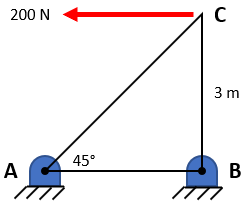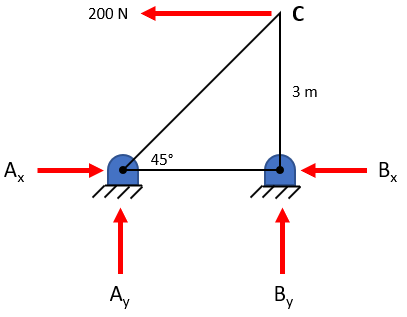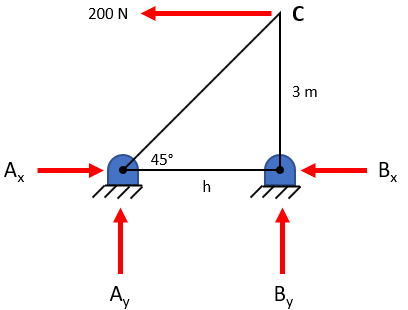## Vertical Force

In the frame below, what is the vertical reaction force at Point A?Hint
Draw the free body diagram:Hint 2
Take the moment about Point B to solve for the unknown:
$$\sum M_B=0=Force \times Distance$$$First, draw a free body diagram and solve for the unknown horizontal leg, $$h$$ :$$tan45^{\circ}=\frac{3m}{h}\rightarrow h=\frac{3m}{tan45^{\circ}}=\frac{3m}{1}=3\:m$$$
Because Points A and B are fixed supports, they have both x and y force components. We can take the moment about Point B to reduce our unknown variables down to 1 ( $$A_x$$ , $$B_x$$ and $$B_y$$ will zero out since their radius vector goes through Point B). Remember, $$Moment=Force \times Distance$$ :
$$\sum M_B=0= 200N(3m)-A_y(3m)-A_x(0)$$$$$A_y(3m)= 200N(3m)\rightarrow A_y=200\:N$$$
200 N